×

×

# socy 2061 Description

##### Description: goes over all key concepts from ch 1-4
6 Pages 10 Views 7 Unlocks
Reviews

STAT EXAM 1 STUDY GUIDE

## Three types of measures of central tendency.Key Concepts:

★ Population-

○ The total set of individuals, objects, groups, or events in which the researchers are interested

★ Sample-

○ A number of cases selected from a population

★ Parameter-

○ It can be regarded as a numerical characteristic of a statistical population or a statistical model.

★ Statistic-

○ A set of procedures used by social scientists to organize, summarize, and communicate numerical information

★ Unit of Analysis-

○ The object of research of individual groups, organizations or social artifacts ■ Hypotheses are usually stated in terms of a relationship between independent and dependent variables

★ Level of Measurement-

○ Include: Nominal measurement, Ordinal Measurement, Interval-Ratio Measurement, and Dichotomous Variables

★ Variable-

○ Something that changes

## The empirical rule states that for a normal distribution.■ Dichotomous variables are a variable that only has two values

■ The Dependent variable is the “effect”

■ Independent variable is the “cause” of the dependent variable

EX) Nominal Variable:

- Using numbers 1 and 2, we can classify female to male

- 1) female 2) male

- Nominal categories cannot be ranked numerically, because the different categories of male and female vary in quality

Variable

Categories

Gender

Female

Male

★ Nominal Measurement-

○ Numbers or other variables are assigned to a set of categories (for the purpose of naming/labeling/classifying observations) We also discuss several other topics like makomé

○ CANNOT be rank-ordered

★ Ordinal Measurement-

○ Numbers are rank-ordered (categories ranging from low to high)

EX) Ordinal Measurement-

- Social class is an example of ordinal measurement

## What are the skewed distribution?- Upper class, middle class, lower class, working class

- A person in the upper class has a higher position than someone in the middle class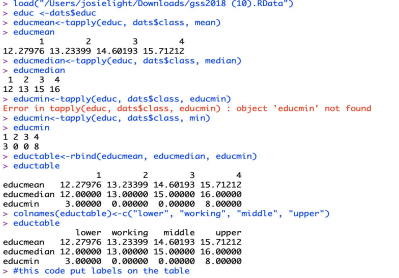R CODE FOR “TAPPLY” FUNCTION WITH CLASSES If you want to learn more check out sw 389 study guide
If you want to learn more check out wayne state university speech pathology

★ Interval-ratio measurement-

○ Measurements are expressed in the same units and equally spaces

○ Interval-ratio values can be rank-ordered

★ Frequency Distributions-

○ A table reporting the number of observations that fall into each category of the variable ○ Contrasting a frequency distribution is usually the first step in the statistical analysis of data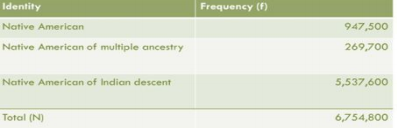★ Measures of Central Tendency-

○ Three types:

■ Mean, median, mode

○ Mode- the category or score with the highest frequency or percentage in the distribution ○ Median- the score that divides the distribution into two equal parts

■ Half the cases are above and half the cases are below

○ Mean- the average of all the total number (arithmetic average)

EX) Mode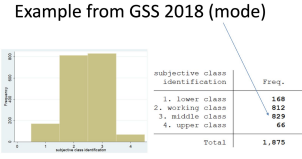If you want to learn more check out What is the meaning of anthropology?

EX) Median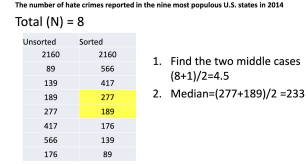★ How to calculate the mean: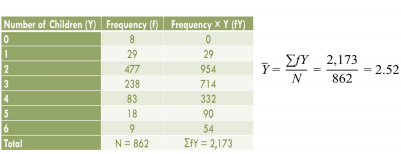We also discuss several other topics like bio 120 exam 3

★ Variance-

○ A measure of variation for interval-ratio and ordinal variables

○ It is the average of the SQUARED deviations from the mean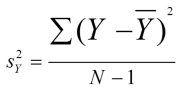If you want to learn more check out jason hafner

★ Standard Deviation-

○ The square root of the variance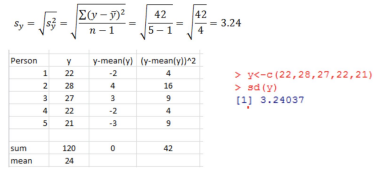★ Skewed Distributions (positive and negative)-

○ Negatively skewed- distribution with a few extremely low values

○ Positively skewed- distribution with extremely high values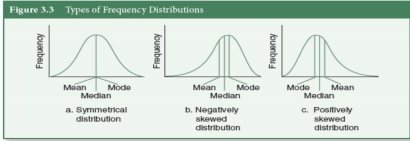R CODE:

- Must install:

- library(psych)

- skew(dats\$age)

★ Percentile-

○ A score below which a specific percentage of the distribution falls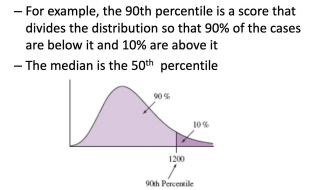★ Interquartile range (IQR)-

○ The width of the middle 50% of the distribution

○ It is defined as the difference between the lower and upper quartiles (Q1 and Q3) ○ IQR can be calculated for interval-ratio and ordinal data

■ Q3= 75%

■ Q1= 25%

○ Quartiles-

■ The median is the second quartile (Q2)

■ The first quartile is the median of the lower half of the observations (Q1)

■ The third quartile is the median of the upper half of the observations (Q3)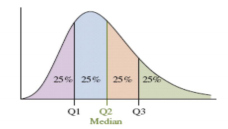★ Pie Chart-

○ A graph showing the differences in frequencies or percentages among the categories of a nominal or an ordinal variable

○ Categories are expressed in a circular graph

★ Bar Graph-

○ A graph showing the differences in frequencies or percentages among the categories of a nominal or an ordinal variable

○ Categories are displayed as rectangles of equal width or their height

★ Histogram-

○ A graph showing the differences in frequencies or percentages among the categories of an interval-ratio variable

○ The categories are displayed as contiguous bars

○ CODE: hist(dats\$age)

★ Empirical Rule-

○ The empirical rule states that for a normal distribution, nearly all of the data will fall within three standard deviations of the mean

o 68% of the observations fall within 1 standard deviation of the mean, that is between x-s and x+s (denoted x(-+)s)

o The height corresponds with sometimes the height or number (relative frequency) o 95% of the observations fall within 2 standard deviations of the mean (x+2s) o All or nearly all observations fall within 2 standard deviations of the mean (x+3s)

Page ExpiredIt looks like your free minutes have expired! Lucky for you we have all the content you need, just sign up here
References: